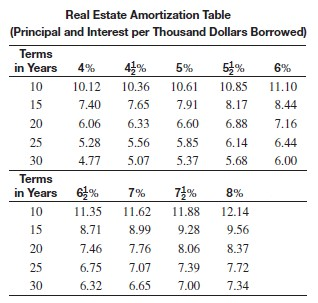/
/
/
87) What is the monthly payment on a 15-year loan of
Not my Question
Flag Content

Question : 87) What is the monthly payment on a 15-year loan of : 2139044

MULTIPLE CHOICE. Choose the one alternative that best completes the statement or answers the question.

Use the real estate amortization table to find the monthly payment for the loan.87) What is the monthly payment on a 15-year loan of \$93,900 if the annual interest rate is 7%?

A) \$ 870.45

B) \$ 844.16

C) \$ 750.26

D) \$ 727.73

88) What is the monthly payment on a 20-year loan of \$68,400 if the annual interest rate is 4 1/2%?

A) \$ 523.26

B) \$ 380.30

C) \$ 414.50

D) \$ 432.97

89) What is the monthly payment on a 10-year loan of \$67,400 if the annual interest rate is 6%?

A) \$ 568.86

B) \$ 748.14

C) \$ 482.58

D) \$ 756.90

90) What is the monthly payment on a 30-year loan of \$63,000 if the annual interest rate is 7 1/2%?

A) \$ 441.00

B) \$ 462.42

C) \$ 465.57

D) \$ 425.25

91) What is the monthly payment on a 20-year loan of \$57,600 if the annual interest rate is 8%?

A) \$ 482.11

B) \$ 550.66

C) \$ 499.97

D) \$ 464.26

92) What is the monthly payment on a 15-year loan of \$101,300 if the annual interest rate is 5%?

A) \$ 1,074.79

B) \$ 774.95

C) \$ 668.58

D) \$ 801.28

93) What is the monthly payment on a 25-year loan of \$93,700 if the annual interest rate is 5 1/2%?

A) \$ 644.66

B) \$ 765.53

C) \$ 575.32

D) \$ 548.15

94) What is the monthly payment on a 30-year loan of \$106,600 if the annual interest rate is 7%?

A) \$ 826.15

B) \$ 708.89

C) \$ 753.66

D) \$ 745.13

95) What is the monthly payment on a 25-year loan of \$101,300 if the annual interest rate is 7 1/2%?

A) \$ 716.19

B) \$ 708.09

C) \$ 816.48

D) \$ 748.61

96) What is the monthly payment on a 30-year loan of \$96,800 if the annual interest rate is 8%?

A) \$ 676.63

B) \$ 744.39

C) \$ 710.51

D) \$ 747.30

Solution 5 (1 Ratings )

Solved
Statistics 1 Year Ago 144 Views